Search datasheet (1.687.043 components) Search fieldPart namePart descriptionAbout site Manufacturers list ChipFindIC search engine AllXrefCross-reference databaseDatasheet: 07N70CP-A (No company)

N-CHENNEL Enhancement-mode Power MOSFET
Download:PDFZIP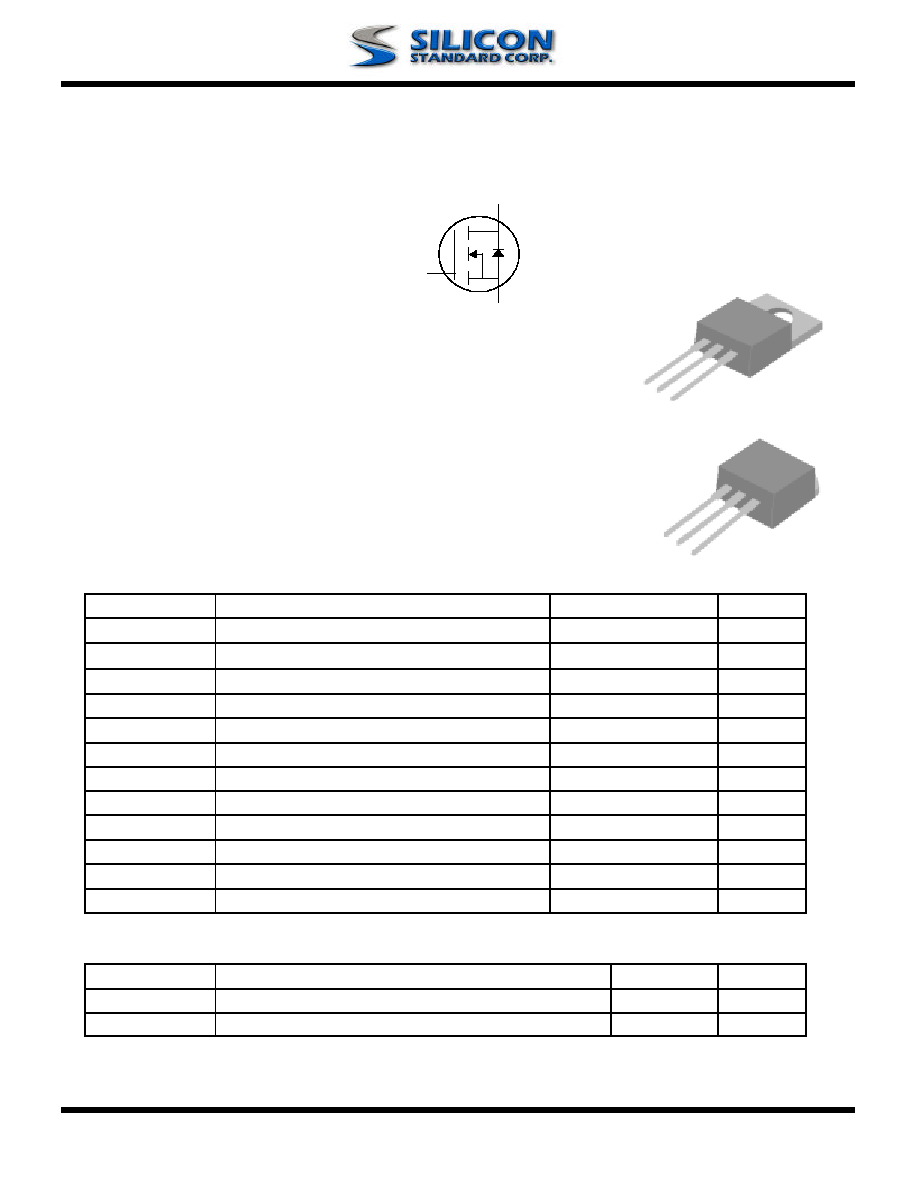www.SiliconStandard.com 1 of 6
N-channel Enhancement-mode Power MOSFET
Dynamic dv/dt rating BV
DSS
675V
Repetitive Avalanche Rated R
DS(ON)
1.2
Fast Switching I
D
7A
Simple Drive Requirement
D
ESCRIPTION
A
BSOLUTE MAXIMUM RATINGS
Symbol Units
V
DS
Drain-Source Voltage V
V
GS
Gate-Source Voltage V
I
D
@
T
C
=2
5�C Continuous Drain Current, V
GS
@ 10V A
I
D
@
T
C
=100
�C Continuous Drain Current, V
GS
@ 10V A
I
DM
Pulsed Drain Current
1
A
P
D
@
T
C
=25
�C Total Power Dissipation W
W
/�C
E
AS
Single Pulse Avalanche Energy
2
mJ
I
AR
Avalanche Current A
E
AR
Repetitive Avalanche Energy mJ
T
STG
T
J
Operating Junction Temperature Rang
e
T
HERMAL DATA
Symbol Value Unit
Rthj-c Thermal Resistance Junction-case Max. 1.4
�C/W
Rthj-a Thermal Resistance Junction-ambient Max. 62
�C/W
140
-55 to 150
�C
Parameter
7
Parameter
Storage Temperature Range -55 to 150
�C
18
89
Linear Derating Factor 0.7
7
7
4.4
Rating
675
The SSM07N70C series is specially designed as
a main switching device
for universal 90~265VAC off-line AC/DC converter applications.
Both TO-220 and TO-262 type provide high blocking voltage to overc
ome
voltage surge and sag in the toughest power system with the best
combination of fast switching,ruggedized design and cost-effectiveness.
The TO-220 and TO-262 package
s are widely preferred for all commercial
and industrial applications. The device is
well suited for switch-mode
power supplies, AC-DC converters and high-current high-speed switching
circuits.
30
G
D
S
TO-262 (R)
G
D
S
TO-220 (P)
G
D
S
SSM07N70CP,R-A
3/21/2005 Rev.2.01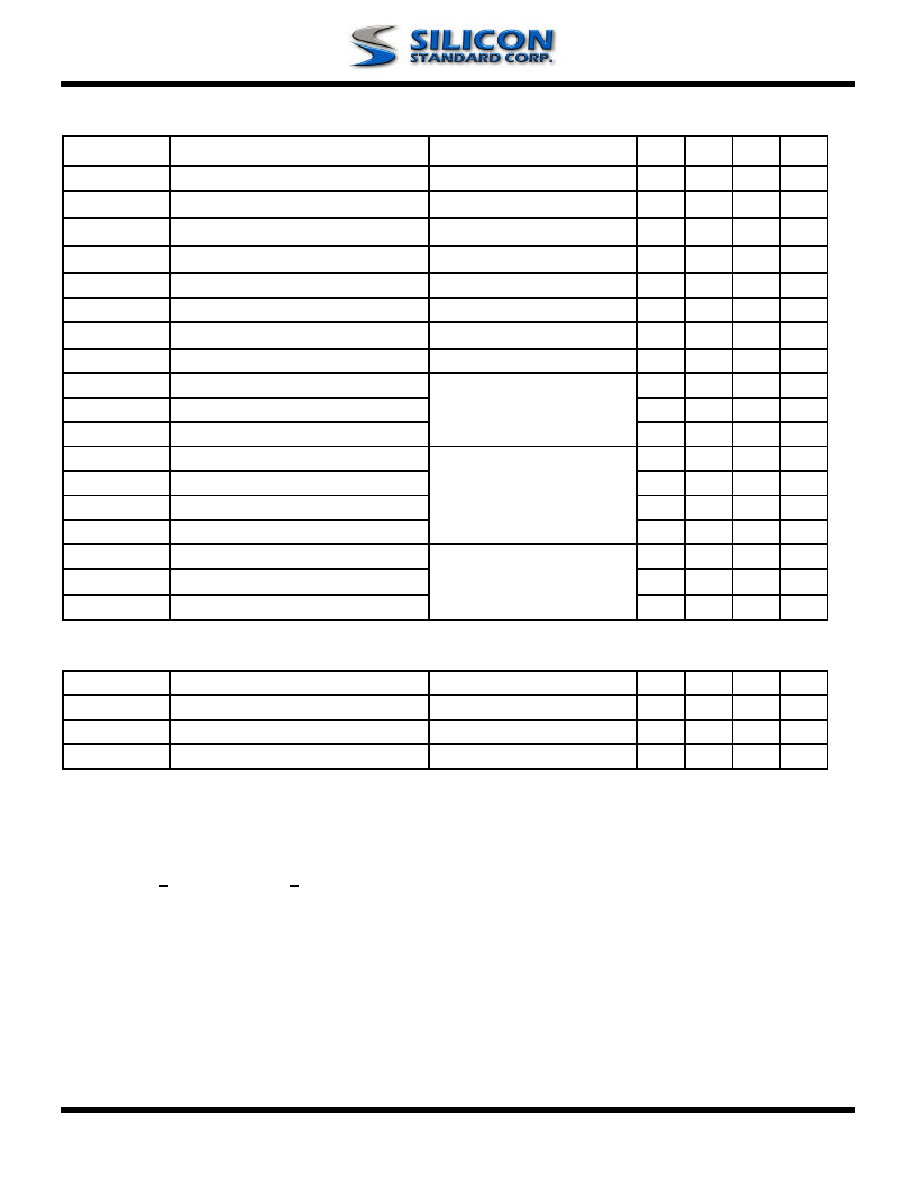www.SiliconStandard.com 2 of 6
Electrical Characteristics@T
j
=25
o
C(unless otherwise specified)
Symbol Parameter Test Conditions Min. Typ. Max. Units
BV
DSS
Drain-Source Breakdown Voltage V
GS
=0V, I
D
=1mA 675 - - V
BV
DSS
/
T
j
Breakdown Voltage Temperature Coefficient
Reference to 25
�C, I
D
=1mA - 0.6 - V/
�C
R
DS(ON)
Static Drain-Source On-Resistance V
GS
=10V, I
D
=3.5A - - 1.2
V
GS(th)
Gate Threshold Voltage V
DS
=V
GS
, I
D
=250uA 2 - 4 V
g
fs
Forward Transconductance V
DS
=10V, I
D
=3.5A - 4.5 - S
I
DSS
Drain-Source Leakage Current (T
j
=25
o
C)
V
DS
=675V, V
GS
=0V - - 10 uA
Drain-Source Leakage Current (T
j
=150
o
C)
V
DS
=480V
,
V
GS
=0V - - 100 uA
I
GSS
Gate-Source Leakage V
GS
=
-
-
nA
Q
g
Total Gate Charge
3
I
D
=7A - 32 - nC
Q
gs
Gate-Source Charge V
DS
=480V - 8.6 - nC
Q
gd
Gate-Drain ("Miller") Charge V
GS
=10V - 9 - nC
t
d(on)
Turn-on Delay Time
3
V
DD
=300V - 17 - ns
t
r
Rise Time I
D
=7A - 15 - ns
t
d(off)
Turn-off Delay Time R
G
=10
,
V
GS
=10V - 35 - ns
t
f
Fall Time R
D
=43
-
18
-
ns
C
iss
Input Capacitance
V
GS
=0V
-
2075
-
pF
C
oss
Output Capacitance
V
DS
=25V
-
120
-
pF
C
Reverse Transfer Capacitance
f=1.0MHz
-
8
-
pF
Source-Drain Diode
Symbol
Parameter
Test Conditions
Min.
Typ. Max. Units
I
S
Continuous Source Current ( Body Diode )
V
D
=V
G
=0V , V
S
=1.5V
-
-
7
A
I
SM
Pulsed Source Current ( Body Diode )
1
-
-
18
A
V
SD
Forward On Voltage
3
T
j
=25
�C, I
S
=7A, V
GS
=0V - - 1.5 V
Notes:
1.Pulse width limited by safe operating area.
2.Starting T
j
=25
o
C , V
DD
=50V , L=5mH , R
G
=25
, I
AS
=7A.
3.Pulse width <300us , duty cycle <2%.

100
30V
SSM07N70CP,R-A
3/21/2005 Rev.2.01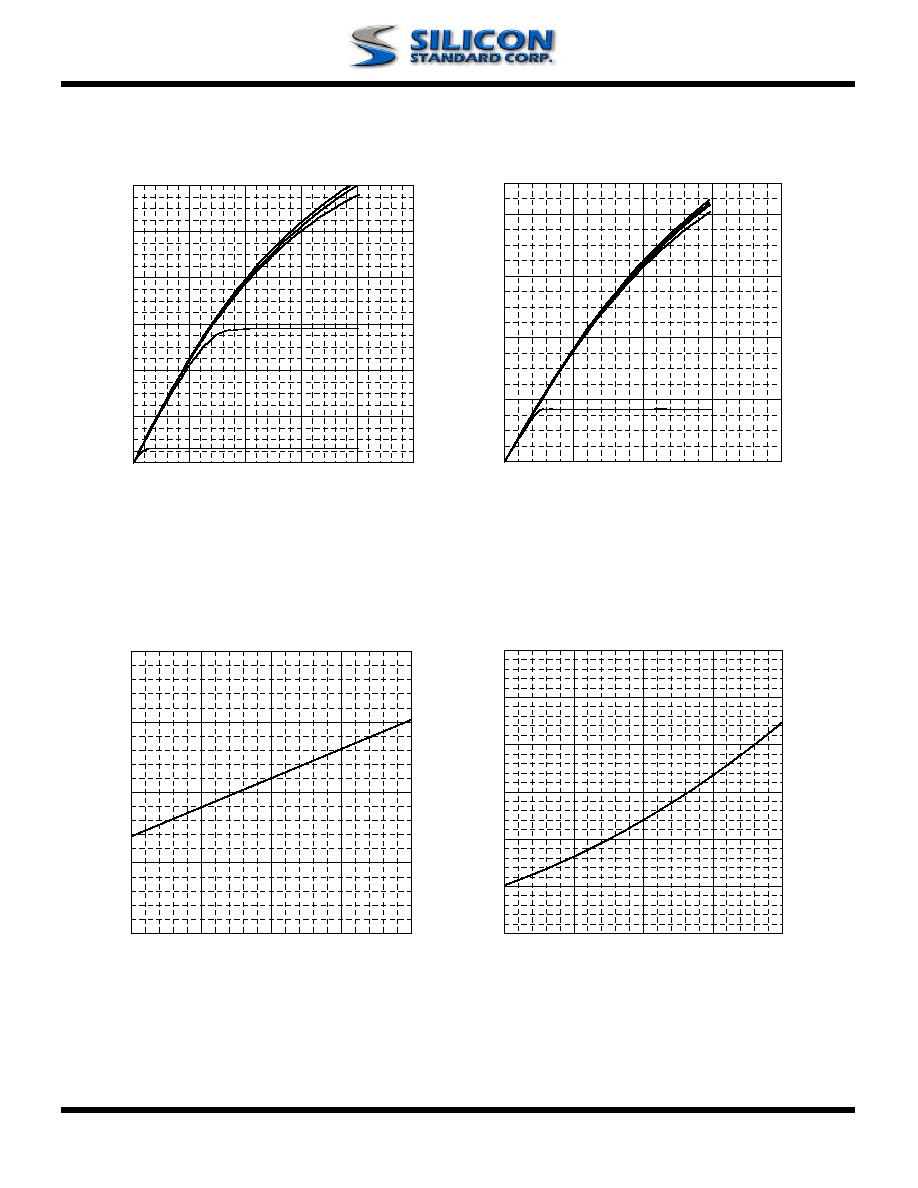www.SiliconStandard.com 3 of 6
Fig 1. Typical Output Characteristics Fig 2. Typical Output Characteristics
Fig 3. Normalized BV
DSS
vs. Junction Fig 4. Normalized On-Resistance
Temperature
vs. Junction Temperature
0
0.5
1
1.5
2
2.5
3
-50
0
50
100
150
T
j
, Junction Temperature (
o
C)
No
rma
l
i
z
e
d
R
DS
(
ON)
V
G
=10V
I
D
=3.5A
0.8
0.9
1
1.1
1.2
-50
0
50
100
150
T
j
, Junction Temperature (
o
C)
No
rma
l
i
z
e
d
B
V
DS
S
(V
)
0
2
4
6
8
10
12
0
5
10
15
20
25
V
DS
, Drain-to-Source Voltage (V)
I
D
,
Dra
i
n C
u
rre
nt
(A
)
T
C
=25
o
C
V
G
=6.0V
V
G
=5.5V
V
G
=5.0V
V
G
=4.0V
V
G
=10V
0
2
4
6
8
0
10
20
30
40
V
DS
, Drain-to-Source Voltage (V)
I
D
,
Dra
i
n C
u
rre
nt
(A
)
T
C
=150
o
C
V
G
=6.0V
V
G
=5.5V
V
G
=5.0V
V
G
=4.0V
V
G
=10V
SSM07N70CP,R-A
3/21/2005 Rev.2.01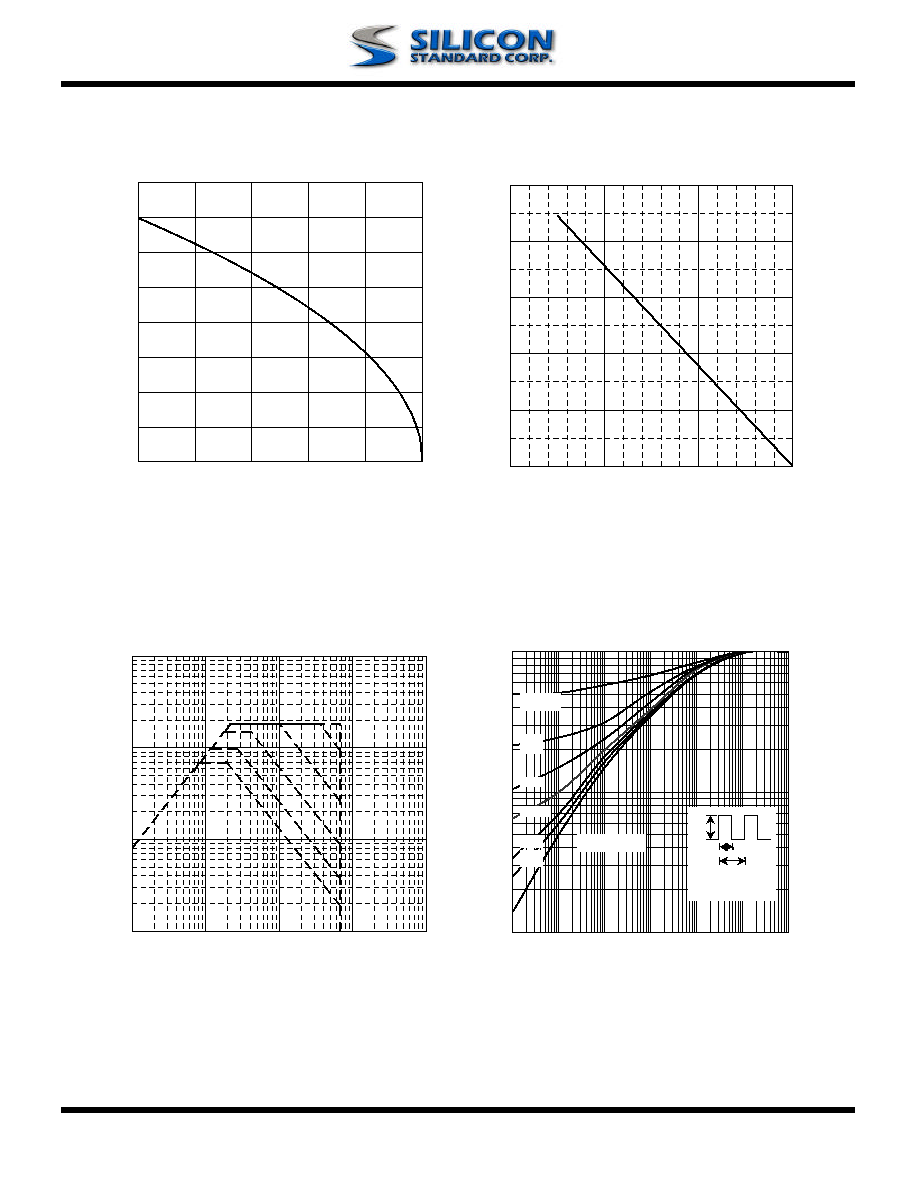www.SiliconStandard.com 4 of 6
Fig 5. Maximum Drain Current vs. Fig 6. Typical Power Dissipation
Case Temperature
Fig 7. Maximum Safe Operating Area Fig 8. Effective Transient Thermal Impedance
0
1
2
3
4
5
6
7
8
25
50
75
100
125
150
T
c
, Case Temperature (
o
C)
I
D
, D
r
a
i
n C
u
r
r
e
nt (
A
)
0
1
10
100
1
10
100
1000
10000
V
DS
(V)
I
D
(A
)
T
c
=25
o
C
Single Pluse
10us
100us
1ms
10ms
100ms
0
20
40
60
80
100
0
50
100
150
Tc , Case Temperature(
o
C)
P
D
(W
)
0.01
0.1
1
0.00001
0.0001
0.001
0.01
0.1
1
10
t , Pulse Width (s)
N
o
r
m
aliz
ed T
h
er
m
a
l Res
pon
s
e
(
R
th
jc
)
P
DM
Duty factor = t/T
Peak T
j
= P
DM
x R
thjc
+ T
C
t
T
0.02
0.01
0.05
0.1
0.2
DUTY=0.5
SINGLE PULSE
SSM07N70CP,R-A
3/21/2005 Rev.2.01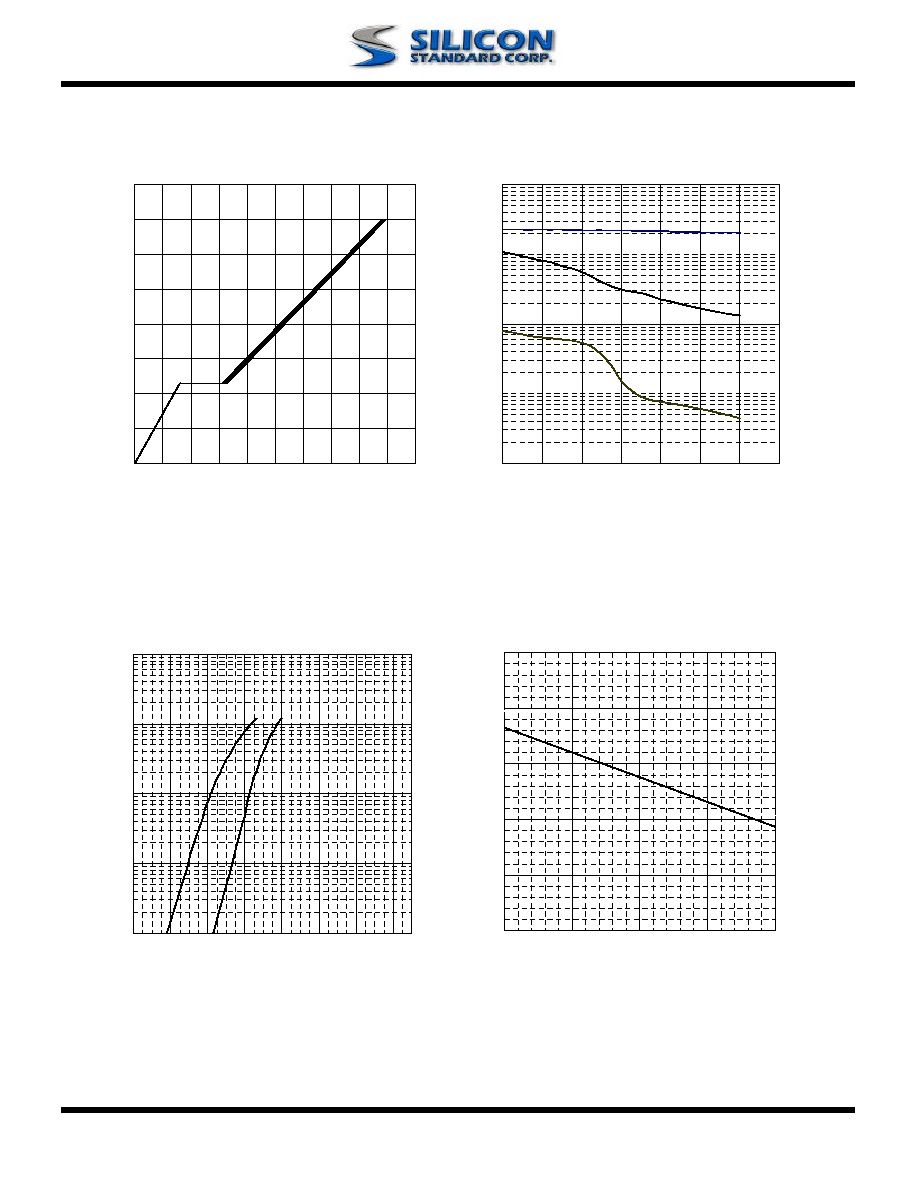www.SiliconStandard.com 5 of 6
Fig 9. Gate Charge Characteristics Fig 10. Typical Capacitance Characteristics
Fig 11. Forward Characteristic of Fig 12. Gate Threshold Voltage vs.
Reverse Diode Junction Temperature
0
1
2
3
4
5
-50
0
50
100
150
T
j
, Junction Temperature (
o
C)
V
GS
(
th
)
(V
)
0.01
0.1
1
10
100
0.1
0.3
0.5
0.7
0.9
1.1
1.3
1.5
V
SD
(V)
I
S
(A
)
T
j
= 25
o
C
T
j
= 150
o
C
0
2
4
6
8
10
12
14
16
0
5
10
15
20
25
30
35
40
45
50
Q
G
, Total Gate Charge (nC)
V
GS
, G
a
te to S
o
u
r
ce Voltage (
V)
I
D
=7A
V
DS
=320V
V
DS
=400V
V
DS
=480V
1
100
10000
1
5
9
13
17
21
25
29
V
DS
(V)
C (
p
F)
f=1.0MHz
Ciss
Coss© 2019 • ICSheet• Contact form• Main page